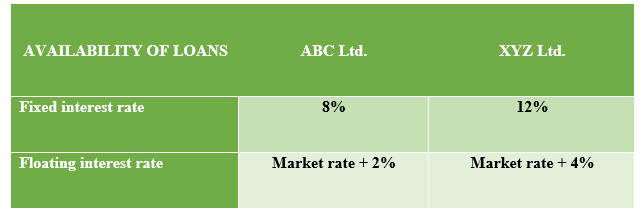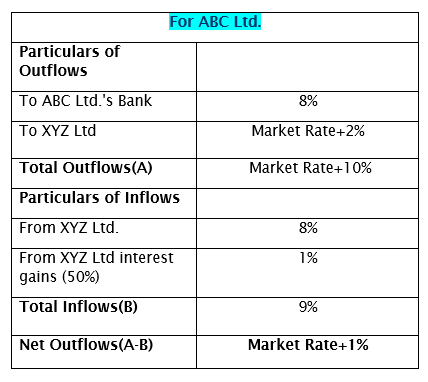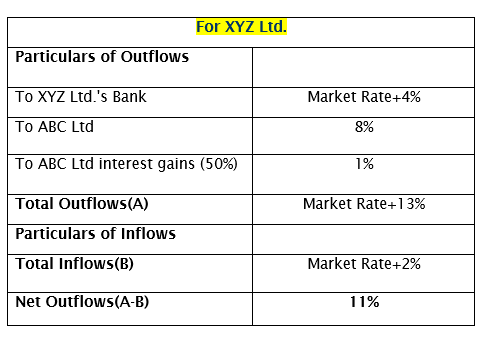# Interest Rate Swap/Example/Valuation## What is an Interest Rate Swap? How to value Interest Rate Swap?

Interest Rate Swap is a forward contract or agreement between two or more parties to exchange interest payment obligations in the future. This agreement clearly defines when the cash flows are to be exchanged and the manner of their calculations by taking into considerations various factors such as predetermined interest rates, foreign exchange rates, predetermined time period or the date and the of notional amount on which interest is to be exchanged.

Basically, there are two types of Interest Rate Swap, a). Fixed Interest Rate Swap, b). Floating Interest rate Swap.

### ⇒Principles of Interest Rate Swap:

In an interest rate swap, one party agrees to pay the interest outgo at a predetermined fixed interest rate on a notional principal and the other party agrees to pay a floating interest rate equal to the cash flows at a predetermined floating interest rate for the same period of time.

Generally, for making interest payments on floating interest rates, LIBOR is taken as the benchmark interest rate. On the other hand, for making interest payments on fixed interest rates, US Treasury Bond is taken as the benchmark interest rate.

### ⇒Benefits of Interest Rate Swap:

• When there are interest rates rise in the market and two companies have different approaches for financing their loans requirements, this swap would enable them in interest rate risk management. As a result, both the parties would end up with lower interest payments which are financially beneficial to them.
• In an interest rate swap agreement there is no requirement of exchange of the principal amount, only the interests are being exchanged.
• Most important benefits of this arrangement are that one can transform a Fixed interest rate loan into a floating interest rate loan and vice-versa.
• In this swap, the principal amount is not exposed to credit risk as the principal amount is not being exchanged, only interest rates are exchanged.

### ⇒ Interest Rate Swap example:

Let’s see an interest rate Swap example and how two companies ABC Ltd. and XYZ Ltd. benefit from this. The following two loan facility is available to these two companies.Analysis:

From the above example, you can see that:

• ABC Ltd. has an advantage of 4% in Fixed interest Rate loans and 2% in Floating interest Rate loans. Therefore, it is clear that ABC Ltd. would enter into Fixed interest Rate loans arrangement with its Bank.
• XYZ Ltd. has opted for Floating Interest Rate loans with its Bank.

#### ⇒ Interest Rate Swap Example with Solution:

Here, both the companies have the advantage of one rate of interest loans to other such as ABC Ltd. has opted for 8% interest rate loans while XYZ Ltd has opted for Market Rate+4% loans with their Banks. Further, this swap agreement’s interest benefits will accrue to the weaker company (XYZ Ltd. here) and later it will surpass the benefits @50% to the stronger company(ABC Ltd.).

In this example, the total net interest rate gains come to 2%. Difference between fixed rate loans i.e. 4% (12-8) and the difference between floating rate interest i.e. 2%(Market rate+ 4-2)

#### ⇒ Interest Rate Swap Agreement:

Since the interest rate swap agreement is resorted to for minimizing the risk of high-interest rate loan repayment, the benefit of rate swapping is to be identified and exercised.

Here, ABC Ltd. will pay to XYZ Ltd. Floating Rate of Interest applicable to ABC Ltd. i.e. Market rate + 2%. On the otherhand, XYZ Ltd. will pay to ABC Ltd. The fixed rate of Interest applicable to ABC Ltd. i.e. 8% and not 12%.Step-1 for ABC Ltd.: ABC Ltd. will pay to its Bank, fixed rate of interest i.e. 8% and to the Bank of XYZ Ltd. Floating interest rate i.e. Market+2%. The total cash flows can be prepared as below.So, loan converted from Fixed rate to Floating rate with lower interest payments. The benefit gained 1%.Step-2 for XYZ Ltd.: XYZ Ltd. will pay to its Bank, Floating interest rate i.e. Market+4% and to the Bank of ABC Ltd. Fixed interest rate i.e. 8%. The total cash flows can be prepared as below.So, loan converted from Floating rate to Fixed rate with lower interest payments. The benefit gained 1%.

Therefore, from the above interest rate swap example & solution you can see that both the companies could manage to save interest outgo by 1% due to this interest rate swap agreement. Is not it very interesting? Did you notice that both companies how beautifully converted their loan repayment net outgo? I mean ABC ltd. started with a fixed rate interest loan and finally ended up with a floating interest rate and for XYZ Ltd. exactly the opposite scenario.

##### ⇒How to value Interest Rate Swap?

Interest Rate Swap Valuation method:

• Firstly, interest amount both receivable and payable under Fixed rate or Floating Rate is to be calculated.
• Secondly, the net interest receivable or payable will have to be discounted to the present value using an appropriate discounting rate.
• The appropriate discount rate is the interest rate under the Floating Rate loan for that period.

The interest rate swap valuation can be done using two methods such as

1. Interest Rate Swap Valuation under Bond Valuation approach;
2. Interest Rate Swap Valuation under Forward Rate Agreement approach;

If you like this post, kindly share with others so that it may help them.